# 连续性方程推导的疑问（微元控制体）

•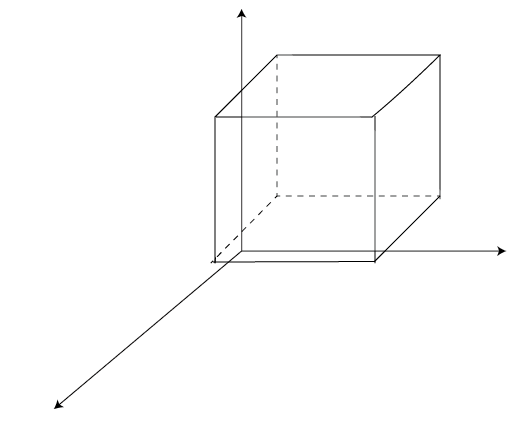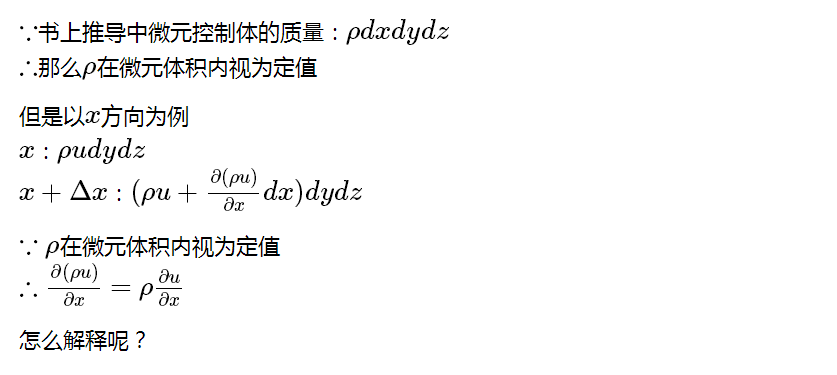注：$\LaTeX$语法可能加载较慢，直接将其弄成图片形式了

• 在控制体内推导方程的时候，所有变量density 和 pressure等等都是空间和时间的函数，不会视为定值。
微元体质量里的density是单元中心的density，如果用泰勒展开只保留一阶的话（假定为线性的），它也等于单元平均值。
所以你的大前提就错了。

• @hangsz density 和 pressure等等都是空间和时间的函数，但是如果density在微元控制体内不为定值，那么微元体的质量应该用积分表示不知是否合理？如果以上式表示质量，继续推导的话，怎么得到书中的微元控制体的连续性方程形式

• 所以你的问题就是为什么$\frac{\partial \rho u}{\partial t}=\rho \frac{\partial u}{t}$？

• x方向流入质量：$(\rho u)|_{x} \Delta y \Delta z$
x方向流出质量：$(\rho u)|_{x+\Delta x} \Delta y \Delta z$
y方向流入质量：$(\rho v)|_{y} \Delta x \Delta z$
y方向流出质量：$(\rho v)|_{y+\Delta y} \Delta x \Delta z$
z方向流入质量：$(\rho w)|_{z} \Delta x \Delta y$
z方向流出质量：$(\rho w)|_{z+\Delta z} \Delta x \Delta y$

于是，
$\frac{\partial \rho}{\partial t}\Delta x \Delta y \Delta z = ((\rho u)|_{x} - (\rho u)|_{x+\Delta x}) \Delta y \Delta z + ((\rho v)|_{y} - (\rho v)|_{y+\Delta y}) \Delta x \Delta z + ((\rho w)|_{z} - (\rho w)|_{z+\Delta z}) \Delta x \Delta y$

两边除以 $\Delta x \Delta y \Delta z$
$\frac{\partial \rho}{\partial t} = \frac{(\rho u)|_{x} - (\rho u)|_{x+\Delta x}}{\Delta x} + \frac{(\rho v)|_{y} - (\rho v)|_{y+\Delta y}} {\Delta y} + \frac{(\rho w)|_{z} - (\rho w)|_{z+\Delta z}} {\Delta z}$

所以
$\frac{\partial \rho}{\partial t} = - \left ( \frac{\partial}{\partial x} (\rho u) + \frac{\partial}{\partial y} (\rho v) + \frac{\partial}{\partial z} (\rho w) \right ) = - \nabla \cdot \rho \textbf U$

对控制体积分，运用高斯定理，可得连续性方程的积分形式
$\frac{\partial}{\partial t} \int \rho dV = - \oint \rho \textbf U \cdot \textbf n dS$

• @cfd-china 其实我的思路是这样的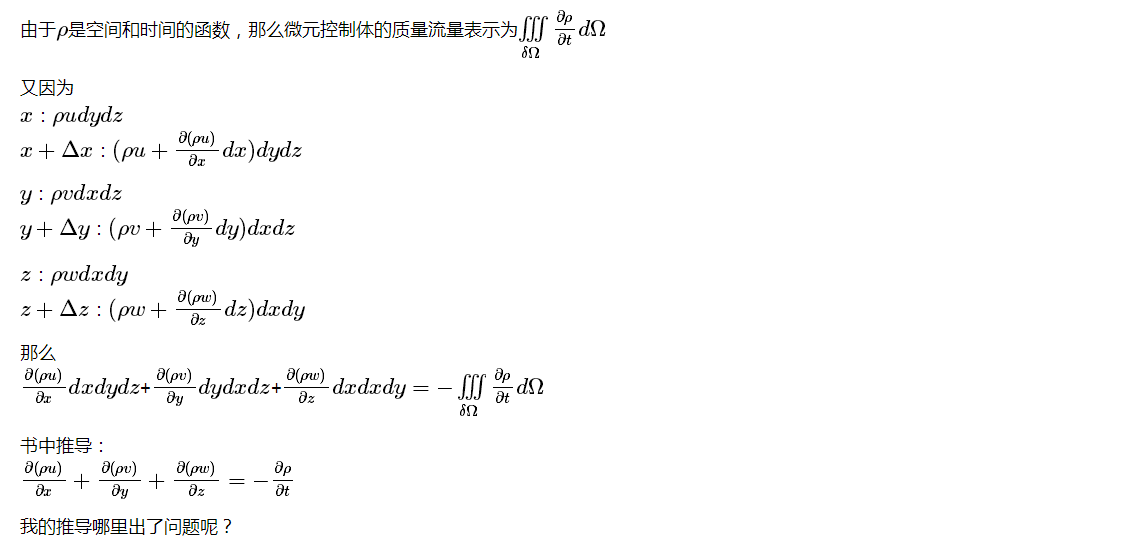我推导不出书中的结论

• @wwzhao 谢谢回复！我的推导有问题吗？

• 你的推倒没问题，只是你没学会变化。
微元体的质量变化率应该是：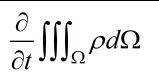由于控制体形状不变，所以和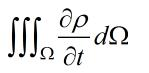是相同的。

而这两个式子都可以化为：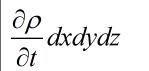关键在于你要理解这里的density是微元中心的密度，它在一阶泰勒展开（线性），也即假设density在单元内是线性变化的时候，等于平均密度，所以三重积分可以写成密度与体积相乘的形式。
偏导符号在三重积分外，比较容易推出。而偏导符号在三重积分内时，由于微分是线性算子，所以之前的密度的线性分布假设也是不受影响的。

吐槽：你们打的公式，全都是符号，可能是我浏览器有问题。

• @hangsz :sunglasses: ,厉害。我的数学基础还是太薄弱。

注：公式全是符号？火狐浏览器容易出现这个问题，应该是渲染不行。chrome和ie浏览器好一点。

• @hangsz 论坛的 MathJax 插件有缺陷，需要刷新页面一次才能显示出来。

• @simthere 你的问题其实可以简化为：为什么 $\int \rho dV = \rho_P \int dV$。（其中 $\rho_P$ 为格心处的流体密度）

下面给出简略证明：

我们假设P为单元格心，$\textbf x_P$ 为P点坐标，那么有如下定义
$\int (\textbf x - \textbf x_P) dV = 0$

于是密度对控制单元的积分为：
$\int \rho dV = \int [\rho_P + (\textbf x - \textbf x_P) \cdot (\nabla \rho_P)] dV = \rho_P \int dV + (\nabla \rho_P) \cdot \int (\textbf x - \textbf x_P) dV = \rho_P \int dV$

同理可以推广到压力、速度等物理量。

• @wwzhao :thanks!